[Documentation] [TitleIndex] [WordIndex]

## Overview

Instead of assuming absolute certainty regarding detected models, this planner views each model as a representation of the real object. One representation may be be better or worse than another, but each is correct to some extent. The planner then uses existing services to get grasps from each representation (the grasp database for database models, the point cluster planner for point clusters, etc.). It then estimates the probability of each grasp's success on the true object by estimating the probability of success of each grasp on each representation, and computing the sum of those probabilities, weighted by the probability that each representation

The following images illustrate how the planner works.

### Setup

The real object is placed on a table and a point cloud is recorded.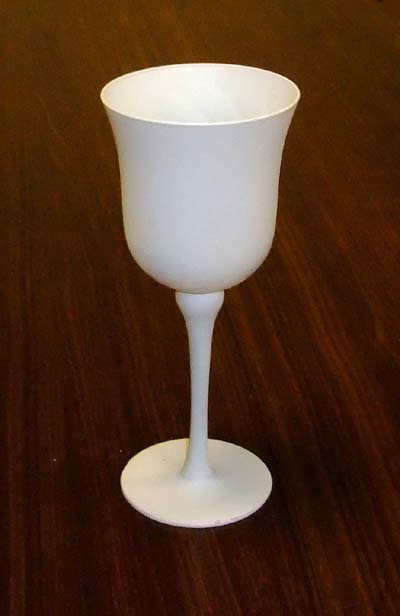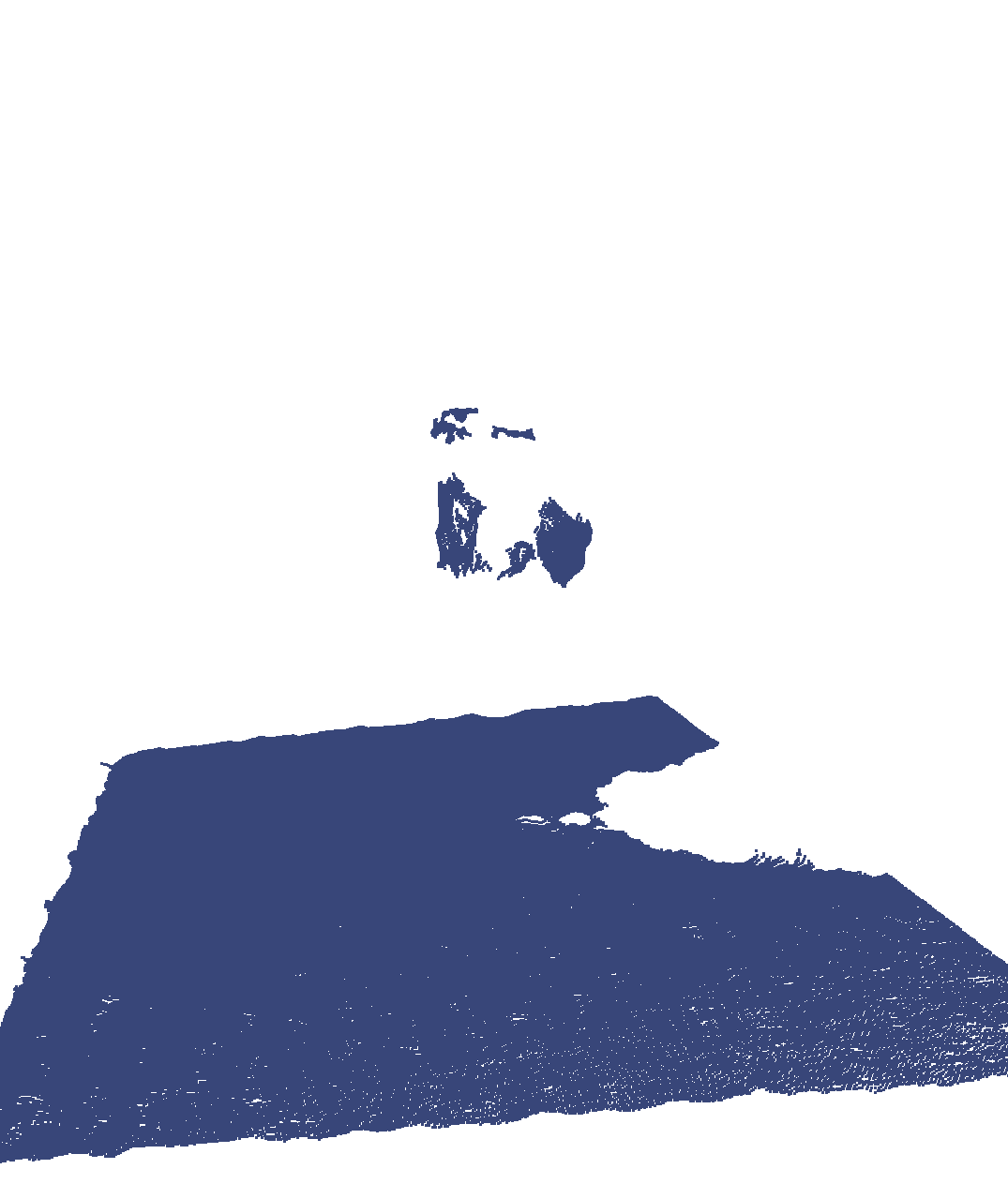### Segmentation and Detection

The table is segmented out, and the salient point cluster is detected for further analysis. Objects are fit to the point cluster, and the top objects are returned (by default, n=5).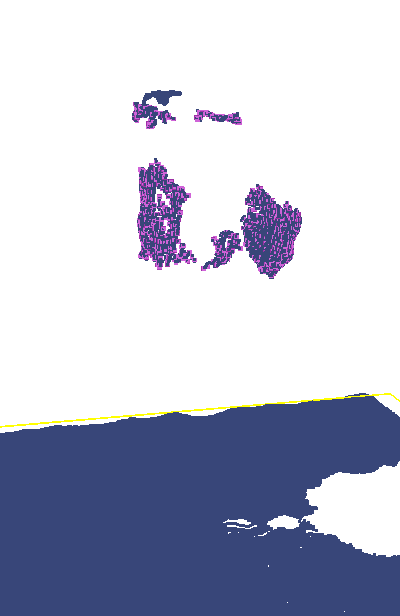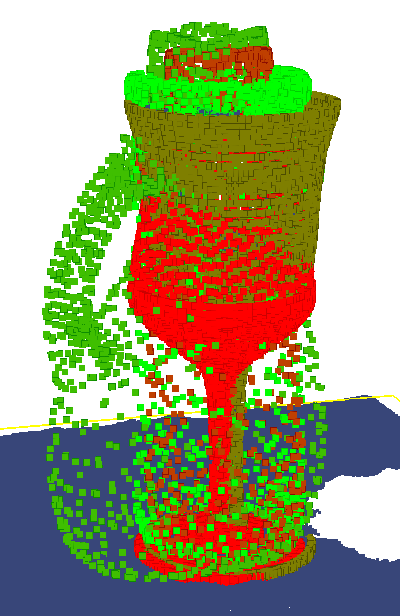### Representations

For each matched object, the grasps computed for that object are retrieved from the database (effectively the same as the database-backed grasp planner). The following image sets show example retrieved grasps from the first object in the list returned by the tabletop object detector (an incorrect object) and the third object (the true object).

The first object is incorrectly detected as a tennis ball can.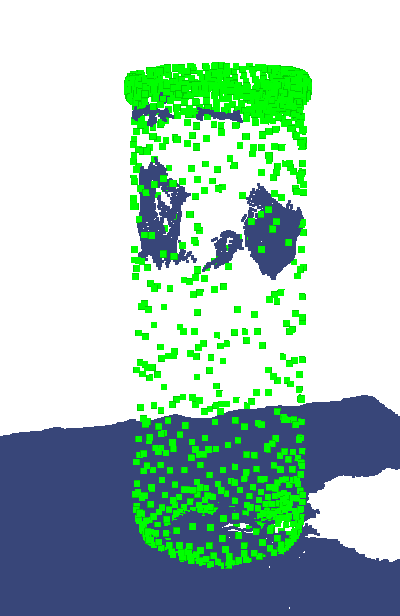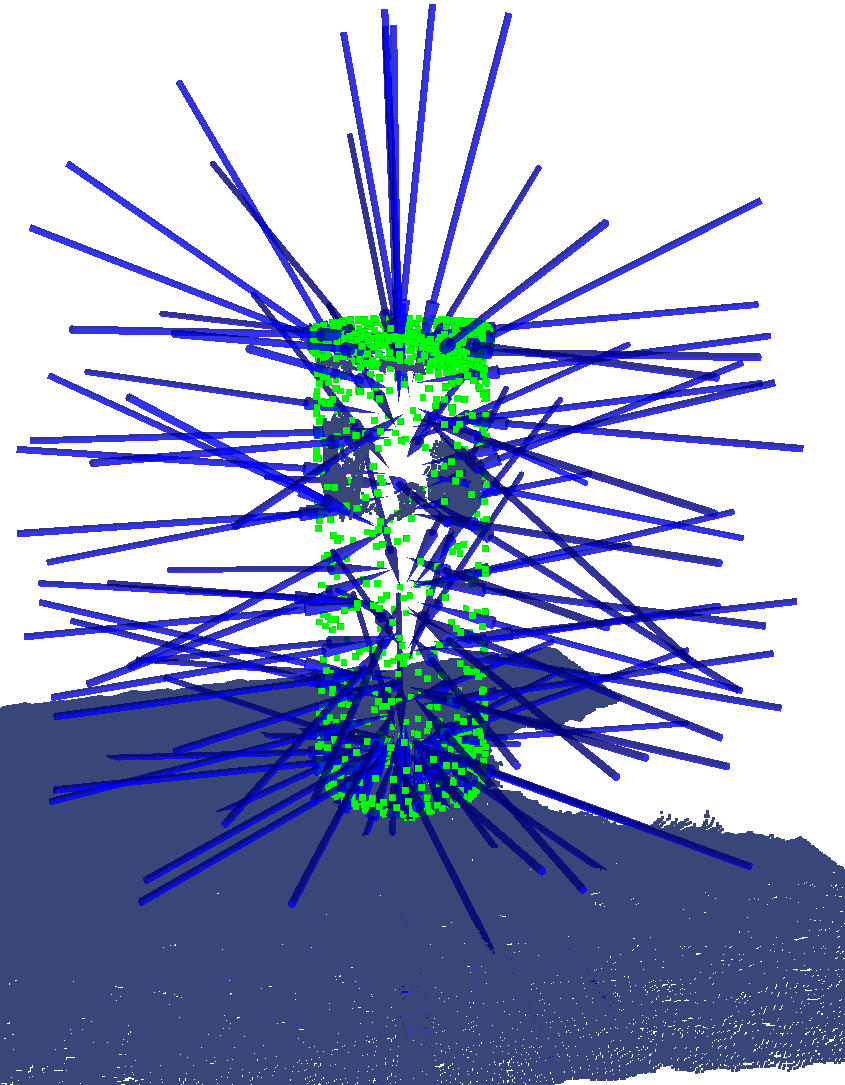The third object is the true object.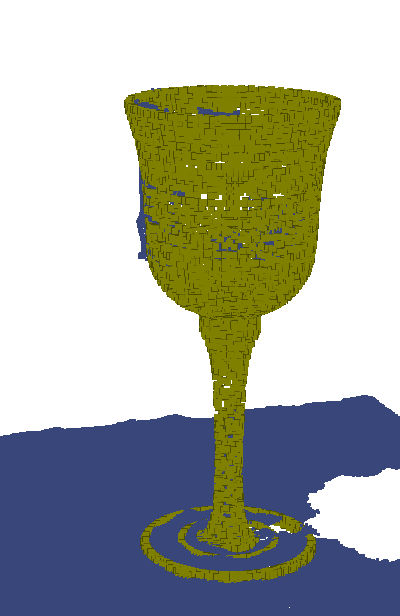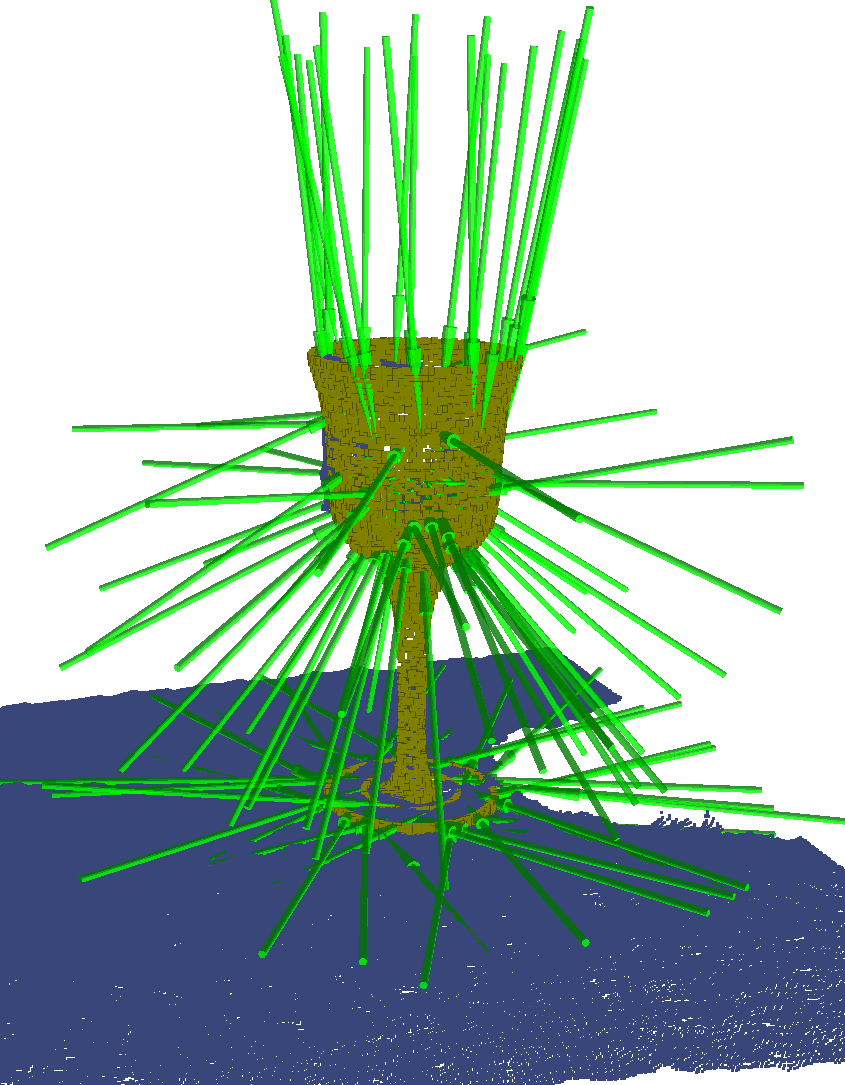Additionally, the point cluster itself is treated as a valid representation of the object (indicating that the object is not in the database), and the point cluster grasp planner is called to retrieve a list of grasps.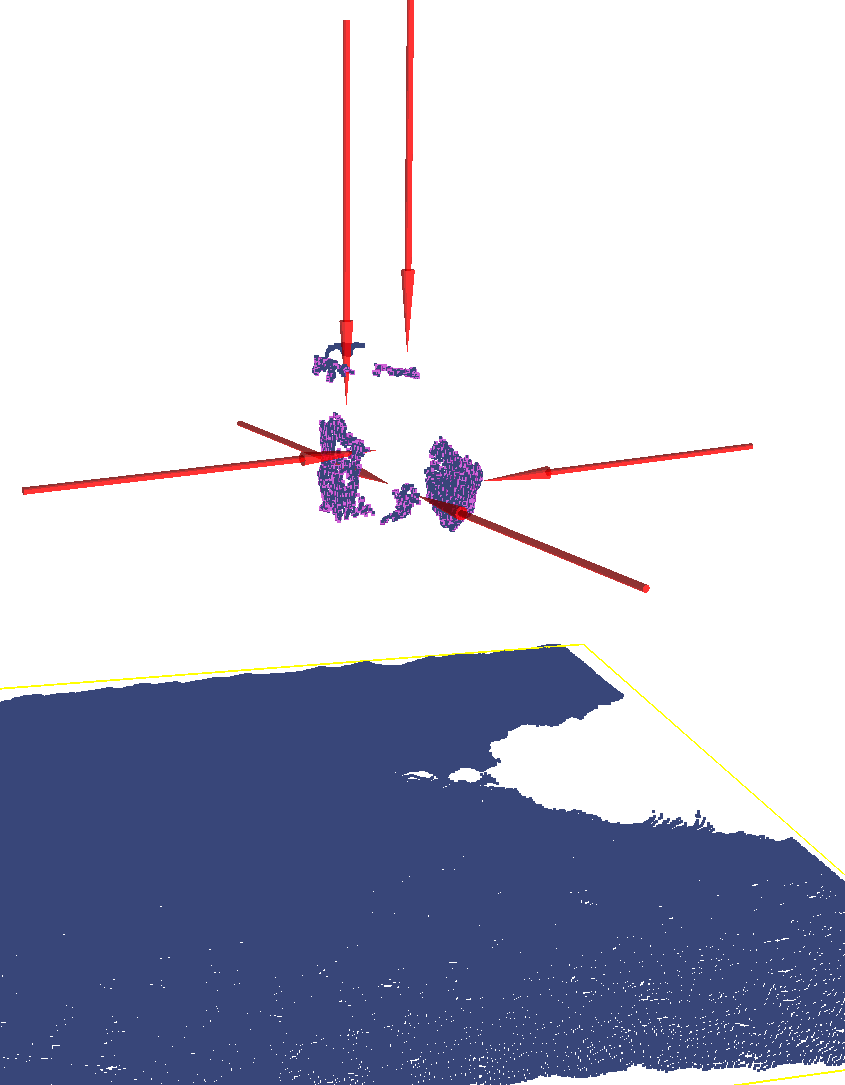### Output

Given the above representations for the objects and associated probabilities for each representation, the planner sorts the list of all the grasps from all representations by the estimated probability of success of each grasp. The probability of success is estimated by computing the probability of success on each object representation, and weighting that by the probability of that representation being the most accurate representation in the list of available representations. The resulting grasps for the example representations are shown below.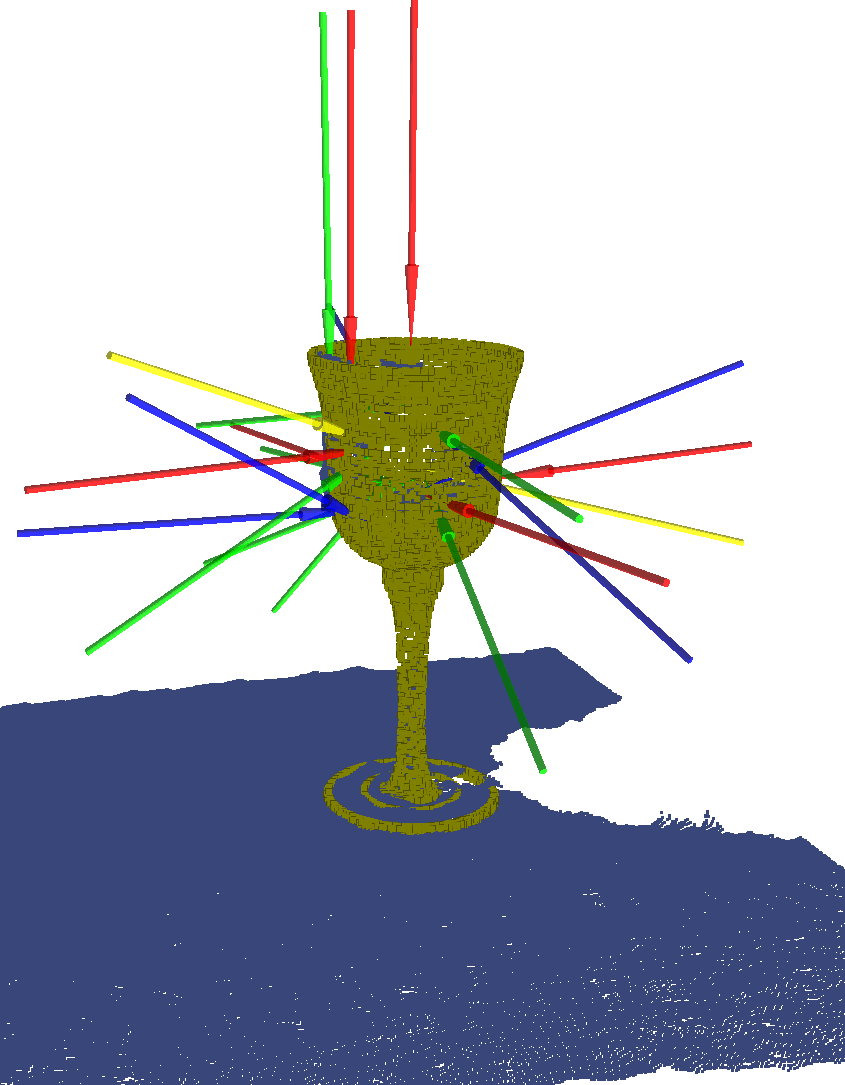2023-01-21 12:55